# Exponential function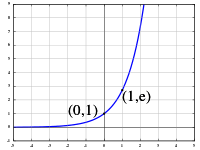The natural exponential function y = ex

In mathematics, an exponential function is a function of the formin which the input variable x occurs as an exponent. A function of the form f(x) = bx + c is also considered an exponential function, and a function of the form f(x) = a·bx can be re-written as f(x) = bx + c by the use of logarithms and so is an exponential function.

Exponential functions are uniquely characterized by the fact that the growth rate of such a function is directly proportional to the value of the function. This proportionality can be expressed by sayingwhere ln b is a constant, and a constant is a quantity that does not change as the variable x changes.

For just one base b this constant factor is equal to 1, and that base is the number e  2.71828...:This equality makes it possible to reduce some questions in mathematical analysis of exponential functions to the analysis of this one exponential function, conventionally called the "natural exponential function" and denoted byThe exponential function models a relationship in which a constant change in the independent variable gives the same proportional change (i.e. percentage increase or decrease) in the dependent variable. The function is often written as exp(x), especially when it is impractical to write the independent variable as a superscript. The exponential function is widely used in [physics], chemistry, engineering, mathematical biology, economics and mathematics.

 Representation ex Inverse ln x Derivative ex Indefinite Integral ex + C

The graph of y = ex is upward-sloping, and increases faster as x increases. The graph always lies above the x-axis but can get arbitrarily close to it for negative x; thus, the x-axis is a horizontal asymptote. The slope of the tangent to the graph at each point is equal to its y-coordinate at that point. The inverse function is the natural logarithm ln(x); because of this, some old texts refer to the exponential function as the antilogarithm.

In general, the variable x can be any real or complex number or even an entirely different kind of mathematical object; see the formal definition below.

## Formal definition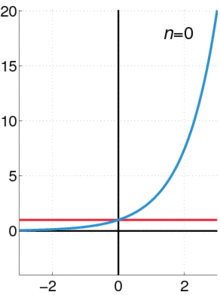The exponential function (in blue), and the sum of the first n + 1 terms of the power series on the left (in red).

The exponential function ex can be characterized in a variety of equivalent ways. In particular it may be defined by the following power series:Using an alternate definition for the exponential function leads to the same result when expanded as a Taylor series.

Less commonly, ex is defined as the solution y to the equationIt is also the following limit:## Overview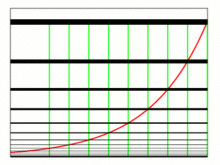The red curve is the exponential function. The black horizontal lines show where it crosses the green vertical lines.

The exponential function arises whenever a quantity grows or decays at a rate proportional to its current value. One such situation is continuously compounded interest, and in fact it was this observation that led Jacob Bernoulli in 1683 to the numbernow known as e. Later, in 1697, Johann Bernoulli studied the calculus of the exponential function.

If a principal amount of 1 earns interest at an annual rate of x compounded monthly, then the interest earned each month is x/12 times the current value, so each month the total value is multiplied by (1 + x/12), and the value at the end of the year is (1 + x/12)12. If instead interest is compounded daily, this becomes (1 + x/365)365. Letting the number of time intervals per year grow without bound leads to the limit definition of the exponential function,first given by Euler. This is one of a number of characterizations of the exponential function; others involve series or differential equations.

From any of these definitions it can be shown that the exponential function obeys the basic exponentiation identity,which is why it can be written as ex.

The derivative (rate of change) of the exponential function is the exponential function itself. More generally, a function with a rate of change proportional to the function itself (rather than equal to it) is expressible in terms of the exponential function. This function property leads to exponential growth and exponential decay.

The exponential function extends to an entire function on the complex plane. Euler's formula relates its values at purely imaginary arguments to trigonometric functions. The exponential function also has analogues for which the argument is a matrix, or even an element of a Banach algebra or a Lie algebra.

## Derivatives and differential equations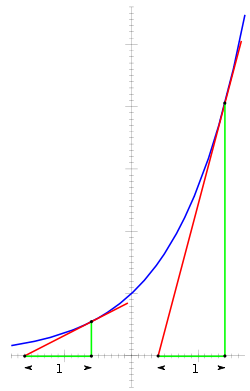The derivative of the exponential function is equal to the value of the function. From any point P on the curve (blue), let a tangent line (red), and a vertical line (green) with height h be drawn, forming a right triangle with a base b on the x-axis. Since the slope of the red tangent line (the derivative) at P is equal to the ratio of the triangle's height to the triangle's base (rise over run), and the derivative is equal to the value of the function, h must be equal to the ratio of h to b. Therefore, the base b must always be 1.

The importance of the exponential function in mathematics and the sciences stems mainly from properties of its derivative. In particular,Proof:That is, ex is its own derivative and hence is a simple example of a Pfaffian function. Functions of the form cex for constant c are the only functions with that property (by the Picard–Lindelöf theorem). Other ways of saying the same thing include:

• The slope of the graph at any point is the height of the function at that point.
• The rate of increase of the function at x is equal to the value of the function at x.
• The function solves the differential equation y′ = y.
• exp is a fixed point of derivative as a functional.

If a variable's growth or decay rate is proportional to its size—as is the case in unlimited population growth (see Malthusian catastrophe), continuously compounded interest, or radioactive decay—then the variable can be written as a constant times an exponential function of time. Explicitly for any real constant k, a function f: RR satisfies f′ = kf if and only if f(x) = cekx for some constant c.

Furthermore, for any differentiable function f(x), we find, by the chain rule:## Continued fractions for ex

A continued fraction for ex can be obtained via an identity of Euler:The following generalized continued fraction for ez converges more quickly:or, by applying the substitution z = x/y:with a special case for z = 2:This formula also converges, though more slowly, for z > 2. For example:## Complex plane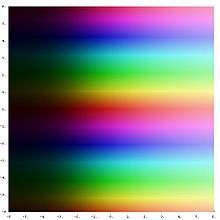Exponential function on the complex plane. The transition from dark to light colors shows that the magnitude of the exponential function is increasing to the right. The periodic horizontal bands indicate that the exponential function is periodic in the imaginary part of its argument.

As in the real case, the exponential function can be defined on the complex plane in several equivalent forms. One such definition parallels the power series definition for real numbers, where the real variable is replaced by a complex one:The exponential function is periodic with imaginary period i and can be written aswhere a and b are real values and on the right the real functions must be used if used as a definition (see also Euler's formula). This formula connects the exponential function with the trigonometric functions and to the hyperbolic functions.

When considered as a function defined on the complex plane, the exponential function retains the properties

•••••for all z and w.

The exponential function is an entire function as it is holomorphic over the whole complex plane. It takes on every complex number excepting 0 as value; that is, 0 is a lacunary value of the exponential function. This is an example of Picard's little theorem that any non-constant entire function takes on every complex number as value with at most one value excepted.

Extending the natural logarithm to complex arguments yields the complex logarithm log z, which is a multivalued function.

We can then define a more general exponentiation:for all complex numbers z and w. This is also a multivalued function, even when z is real. This distinction is problematic, as the multivalued functions log z and zw are easily confused with their single-valued equivalents when substituting a real number for z. The rule about multiplying exponents for the case of positive real numbers must be modified in a multivalued context:

(ez)w
ezw
, but rather (ez)w
= e(z + 2πin)w
multivalued over integers n

See failure of power and logarithm identities for more about problems with combining powers.

The exponential function maps any line in the complex plane to a logarithmic spiral in the complex plane with the center at the origin. Two special cases might be noted: when the original line is parallel to the real axis, the resulting spiral never closes in on itself; when the original line is parallel to the imaginary axis, the resulting spiral is a circle of some radius.

### Computation of ab where both a and b are complex

Main article: Exponentiation

Complex exponentiation ab can be defined by converting a to polar coordinates and using the identity (eln(a))b
= ab
:However, when b is not an integer, this function is multivalued, because θ is not unique (see failure of power and logarithm identities).

## Matrices and Banach algebras

The power series definition of the exponential function makes sense for square matrices (for which the function is called the matrix exponential) and more generally in any Banach algebra B. In this setting, e0 = 1, and ex is invertible with inverse ex for any x in B. If xy = yx, then ex + y = exey, but this identity can fail for noncommuting x and y.

Some alternative definitions lead to the same function. For instance, ex can be defined asOr ex can be defined as f(1), where f: RB is the solution to the differential equation f ′(t) = xf(t) with initial condition f(0) = 1.

## Lie algebras

Given a Lie group G and its associated Lie algebra, the exponential map is a mapG satisfying similar properties. In fact, since R is the Lie algebra of the Lie group of all positive real numbers under multiplication, the ordinary exponential function for real arguments is a special case of the Lie algebra situation. Similarly, since the Lie group GL(n,R) of invertible n × n matrices has as Lie algebra M(n,R), the space of all n × n matrices, the exponential function for square matrices is a special case of the Lie algebra exponential map.

The identity exp(x + y) = exp(x)exp(y) can fail for Lie algebra elements x and y that do not commute; the Baker–Campbell–Hausdorff formula supplies the necessary correction terms.

## Similar properties of e and the function ez

The function ez is not in C(z) (i.e., is not the quotient of two polynomials with complex coefficients).

For n distinct complex numbers {a1, …, an}, the set {ea1z, …, eanz} is linearly independent over C(z).

The function ez is transcendental over C(z).

## exp and expm1

Some calculators provide a dedicated exp(x) function designed to provide a higher precision than achievable by using ex directly.

Based on a proposal by William Kahan and first implemented in the Hewlett-Packard HP-41C calculator in 1979, some scientific calculators, computer algebra systems and programming languages (for example C99) support a special exponential minus 1 function alternatively named E^X-1, expm1(x), expm(x), or exp1m(x) to provide more accurate results for values of x near zero compared to using exp(x)-1 directly. This function is implemented using a different internal algorithm to avoid an intermediate result near 1, thereby allowing both the argument and the result to be near zero. Similar inverse functions named lnp1(x), ln1p(x) or log1p(x) exist as well.[nb 1]

## Notes

1. For a similar approach to reduce round-off errors of calculations for certain input values see trigonometric functions like versine, vercosine, coversine, covercosine, haversine, havercosine, hacoversine, hacovercosine, exsecant and excosecant.

## References

1. Goldstein, Lay; Schneider, Asmar (2006). Brief calculus and its applications (11th ed.). Prentice–Hall. ISBN 0-13-191965-2.
2. Courant; Robbins (1996). Stewart, ed. What is Mathematics? An Elementary Approach to Ideas and Methods (2nd revised ed.). Oxford University Press. p. 448. ISBN 0-13-191965-2. This natural exponential function is identical with its derivative. This is really the source of all the properties of the exponential function, and the basic reason for its importance in applications…
3. Converse; Durrell (1911). Plane and spherical trigonometry. C. E. Merrill Co. p. 12. Inverse Use of a Table of Logarithms; that is, given a logarithm, to find the number corresponding to it, (called its antilogarithm)…
4. Rudin, Walter (1987). Real and complex analysis (3rd ed.). New York: McGraw-Hill. p. 1. ISBN 978-0-07-054234-1.
5. Eli Maor, e: the Story of a Number, p.156.
6. John J O'Connor; Edmund F Robertson. "The number e". School of Mathematics and Statistics. University of St Andrews, Scotland. Retrieved 2011-06-13.
7. "A.2.2 The exponential function." L. Lorentzen and H. Waadeland, Continued Fractions, Atlantis Studies in Mathematics, page 268.
8. Ahlfors, Lars V. (1953). Complex analysis. McGraw–Hill Book Company, Inc.
9. HP 48G Series – Advanced User's Reference Manual (AUR) (4 ed.). Hewlett-Packard. December 1994 . HP 00048-90136, 0-88698-01574-2. Retrieved 2015-09-06.
10. HP 50g / 49g+ / 48gII graphing calculator advanced user’s reference manual (AUR) (2 ed.). Hewlett-Packard. 2009-07-14 . HP F2228-90010. Retrieved 2015-10-10.Searchable PDF
11. Beebe, Nelson H. F. (2002-07-09). "Computation of expm1 = exp(x)−1" (PDF). 1.00. Salt Lake City, Utah, USA: Department of Mathematics, Center for Scientific Computing, University of Utah. Retrieved 2015-11-02.